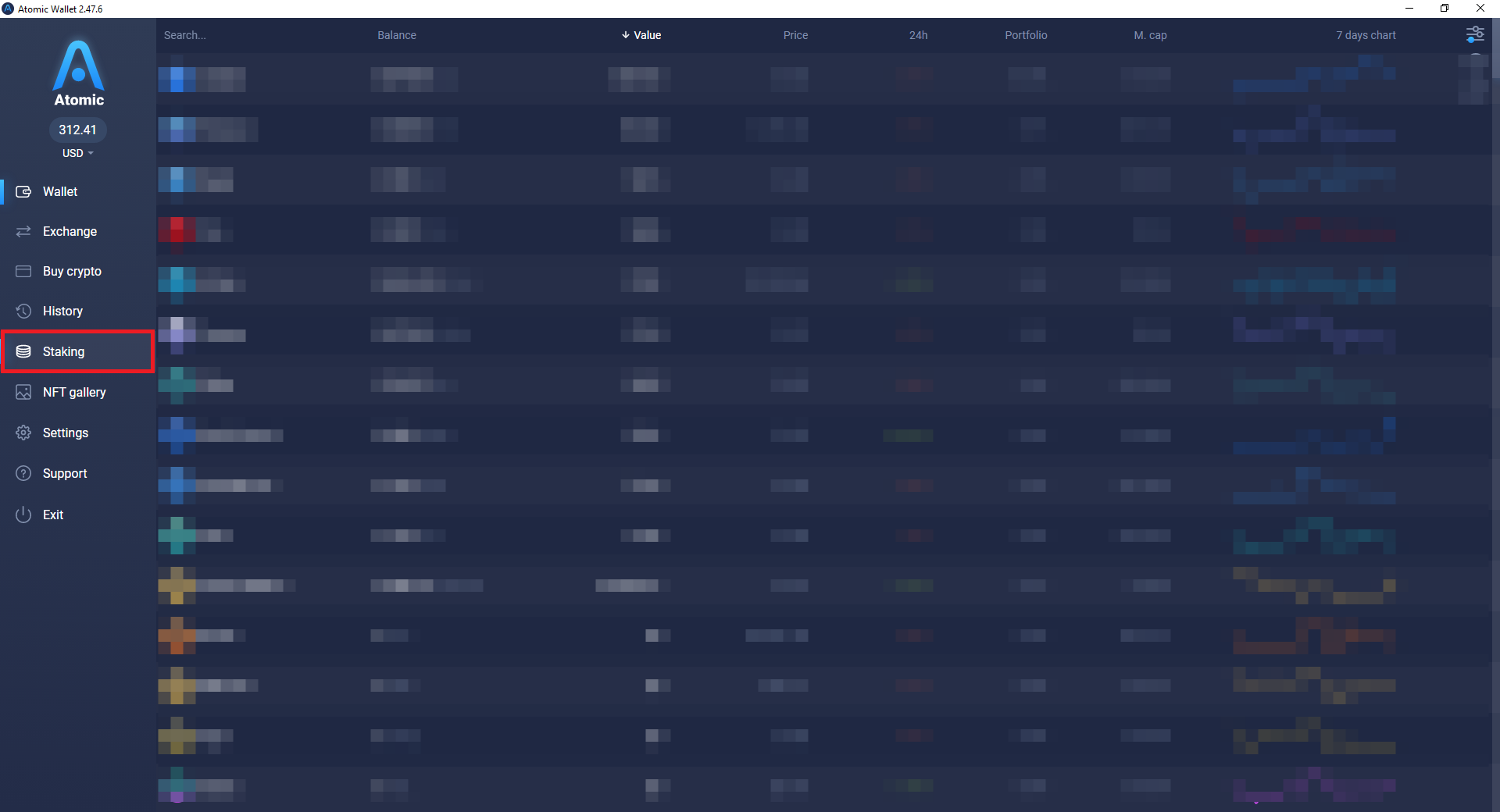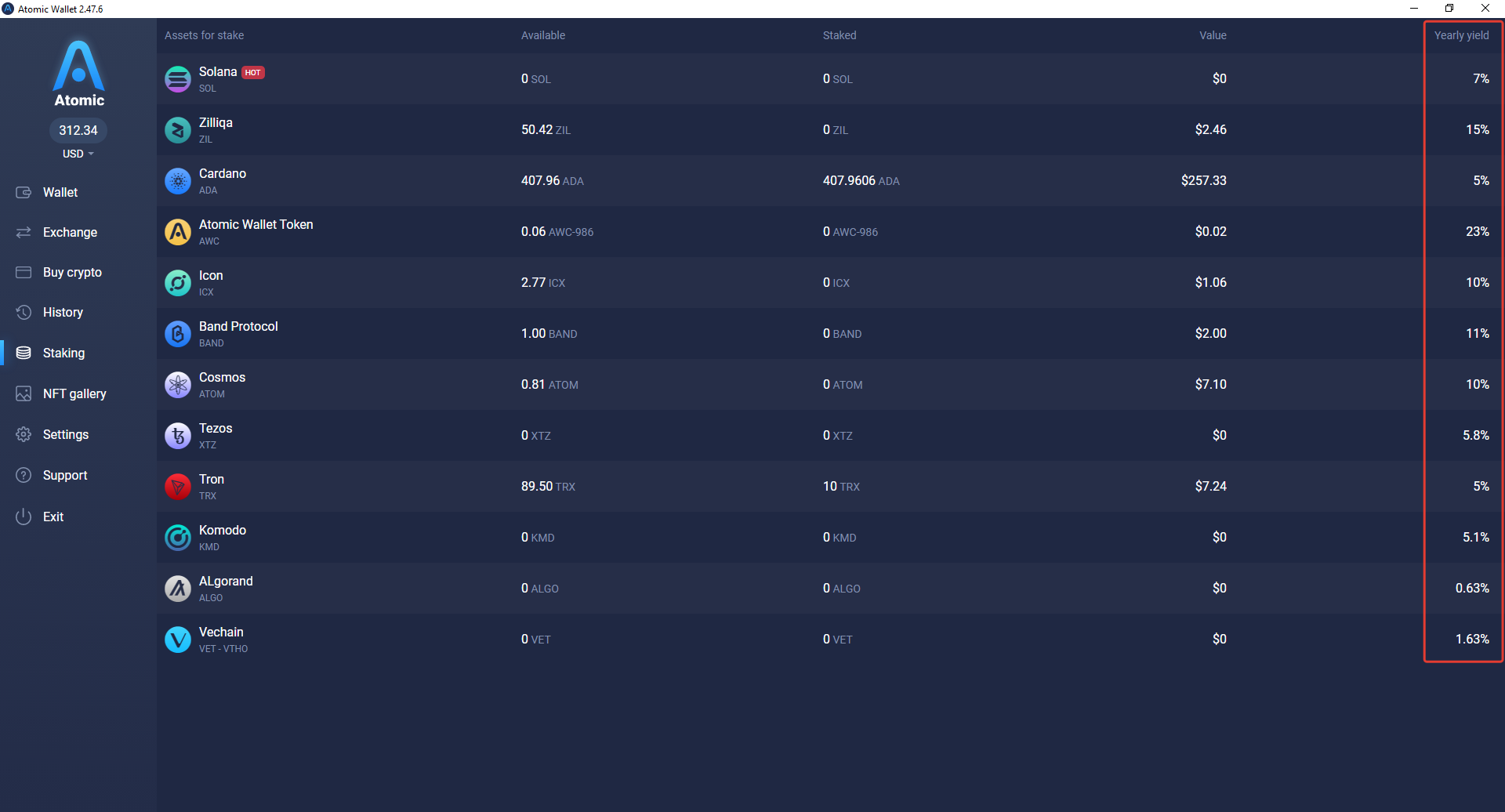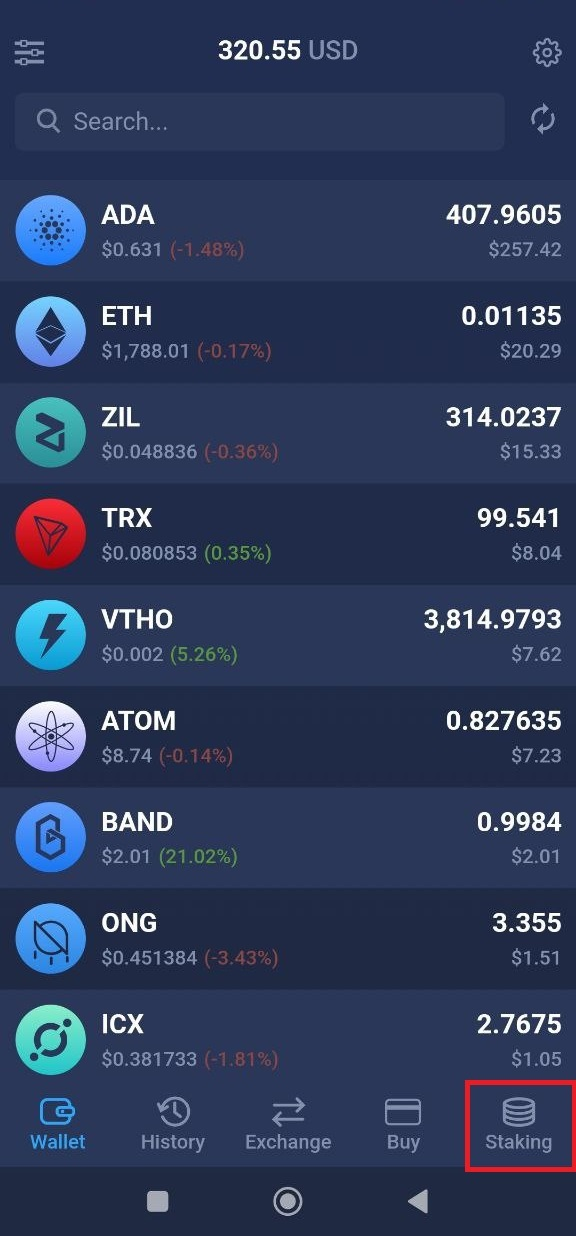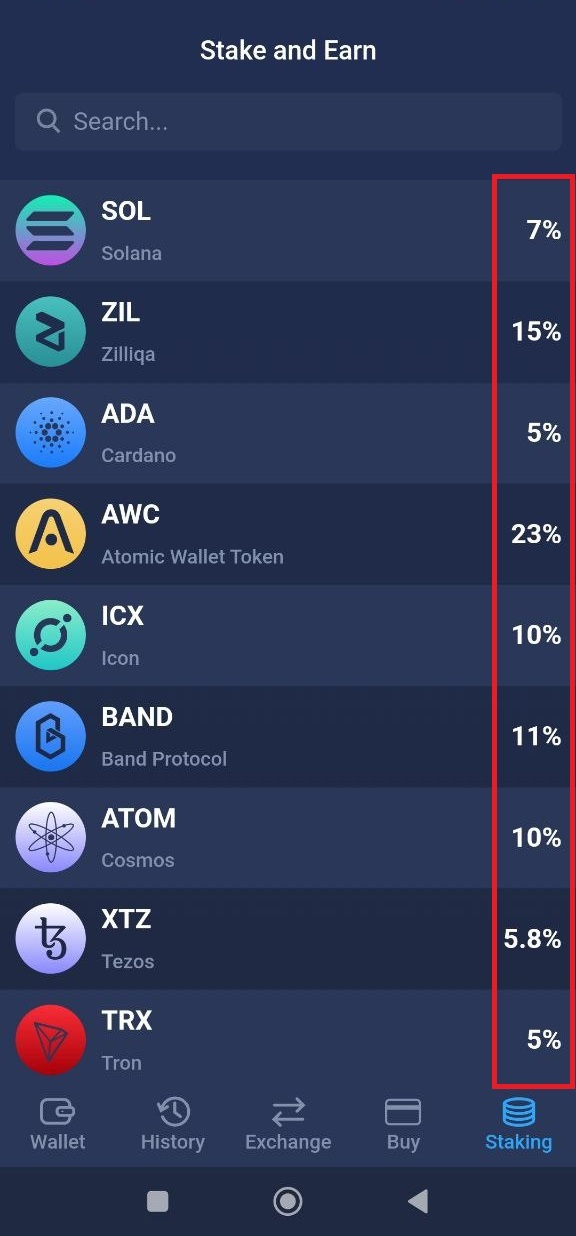# What is APY?

### What is APY?

APY, or Annual Percentage Yield is the estimated percentage of annual return on your staking investment. In other words, the amount of money, or interest, you earn on a certain staked asset over one year.

### How does Atomic Wallet calculate the APY for staking assets?

APY of a staked asset in Atomic Wallet is an average approximation. We are taking data from all available staking pools and calculate the average APY based on the APY of each pool. For example, if a coin has two available staking pools, one offering a 20% APY and the other - a 10% APY, the average APY for this coin will be 15%.

### What is the APY of my staked coins?

APY depends on many factors of a particular blockchain: inflation rate of the network, number of found blocks, number of validators, and amount of staked coins. Remember, that APY is an approximate amount and it may change several times during the staking period of your asset.

Rewards are distributed differently depending on the asset you staked. For example, with some assets, staking works on a mined block per block cycle, while others use epoch, cycles, and so on.

All of these aspects lead to fluctuation of your staking APY. Because of that, the average APY that is displayed in the wallet may differ from the APY you are getting with your staking.

### How do I calculate the amount of rewards I'll be receiving?

There is a specific formula to calculate how much you'll be receiving when staking a specific coin. The formula goes as follows: N*X/Y.

N is the amount you staked, X is the APY and Y is the rewards generation period, i.e., how often you receive your rewards. Let's make it more clear by looking at some examples.

First, let's take ZIL with daily reward generation (meaning 365 payouts) and an APY of 15%. Let's say you stake 1000 ZIL. We have 1000*0.15/365 = 0.41 ZIL. That is how much ZIL you'll get every day. Let's look at another asset with a different APY and payout period, like ATOM.

ATOM has an APY of 10% and you get your rewards weekly (that's 52 payouts). We'll go with 5000 ATOM. Here we have 5000*0.10/52 = 9.61 ATOM. This means you'll be getting 9.61 ATOM every week.

### Do crypto assets have a compound interest?

Compound interest is a term most often used in relation to bank deposits. It is the process of adding interest to the initial sum of the deposit. In cryptocurrency terms that would mean the process of adding staking rewards to your initial staked amount. Some crypto assets do indeed have this feature. For example SOL, ALGO, or ADA. But this is more of an exception, rather than a rule. With most crypto assets, you are only receiving rewards on the amount you staked initially. For more information about the distribution of rewards for different assets consult this guide

### How can I see the APY of the assets that I am staking?

1. Open the wallet and click Staking on the left sidebar.2. The APYs will be displayed in the last column to the right1. Select the Staking tab in the bottom panel2. The APYs will be displayed to the right of the asset name